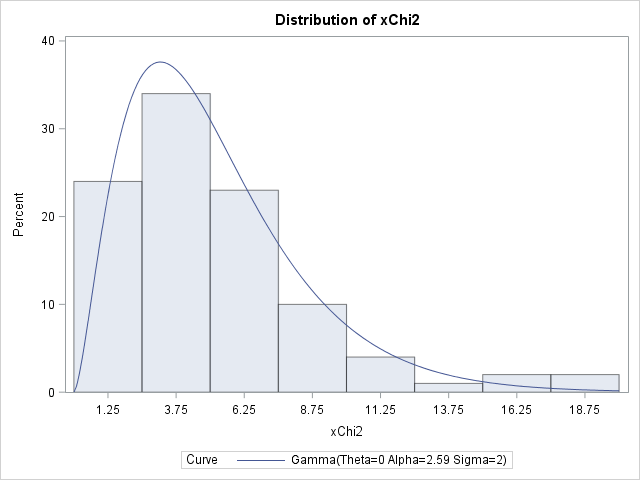Why isn't the chi-square distribution supported in PROC UNIVARIATE?

That is an excellent question. I remember asking a similar question when I first started learning SAS. In addition to the chi-square distribution, I wondered why the UNIVARIATE procedure does not support the F distribution. These are common distributions that appear often in statistics textbooks. Why aren't they among the list of distributions that PROC UNIVARIATE can fit?

There are three ways to address the question. One is philosophical, the other graphical, the third is computational. (Spoiler Alert: PROC UNIVARIATE actually does support fitting the chi-square distribution!)

### Data distributions versus sampling distributions

The HISTOGRAM statement in UNIVARIATE procedure can fit many continuous parametric distributions to observed data. It supports standard distributions such as the exponential, lognormal, normal, and Weibull distributions, as well as some less-common distributions such as the bounded and unbounded Johnson distributions. Other SAS procedures can fit additional distributions. So why doesn't UNIVARIATE support the chi-square and F distributions?

The philosophical answer is that PROC UNIVARIATE is designed to model distributions of observed data, whereas the chi-square and F distributions arise naturally as the sampling distribution of a statistic.

When you model real data (size, weight, pressure, time-to-failure, and so forth), you should choose a modeling distribution that plausibly describes the population from which a random sample was drawn. This often leads to models such as the normal, lognormal, Weibull, and so forth. It usually doesn't make sense to say, "I think I'll model these data by using a chi-square distribution." The chi-square and F distributions describe (asymptotic) sampling distributions of statistics. We don't usually fit their parameters; the parameters represent degrees of freedom and are determined by the sample size, the number of groups, and similar considerations.

Consequently, the philosophical answer is that PROC UNIVARIATE does not support these distributions because they are not common models for observed data.

### Overlaying a distribution on a histogram

There is another way to interpret the question. Many statistical programmers use PROC UNIVARIATE as an easy way to overlay a parametric density curve on a histogram. Therefore the programmer might be asking the practical question, "how can I overlay a chi-square (or F) distribution on a histogram." For example, in a simulation study, you might want to overlay an asymptotic density curve on a histogram of Monte Carlo estimates.

I have previously described how to overlay a custom density curve on a histogram. All that is required is the ability to evaluate the probability density function (PDF) for the distribution. The PDF function in SAS can evaluate the chi-square and F distributions, so it is straightforward to overlay these distributions on a histogram.

### Fitting a chi-square distribution to data

There is a third answer to the question. The chi-square distribution with d degrees of freedom is equivalent to a gamma(d/2, 2) distribution. PROC UNIVARIATE supports fitting the gamma distribution, so you can actually fit a chi-square model as a special case of the gamma distribution. As mentioned in the first section, you probably wouldn't do this for real data, but you might do it as part of a simulation study.

The following program simulates data from a chi-square distribution with 5 degrees of freedom, then fits a gamma density to the data. Use the SCALE=2 option to restrict the gamma family to the chi-square family. Use SHAPE=EST to compute a maximum likelihood estimate of the shape parameter, as follows:

```data ChiSq(keep=xChi2); call streaminit(54321); do i = 1 to 100; xChi2 = rand("chisq", 5); /* 5 degrees of freedom */ output; end; run;   /* use fact that chiSquare(d) = gamma(d/2, 2) to fit chi-square to data */ proc univariate data=ChiSq; histogram xChi2 / gamma(shape=est scale=2); /* fix scale=2, estimate shape parameter */ run;```The estimate of the shape parameter is 2.59. Double this value to obtain an estimate for the degree-of-freedom parameter, which results in the estimate d = 5.18. This is close to the parameter value (5) that was used to simulate the data.

By the way, you can use a similar trick to fit a uniform distribution by using PROC UNIVARIATE. The uniform distribution is a sub-family of the beta distribution, with ALPHA=1 and BETA=1.

As far as I know, there is no way to use PROC UNIVARIATE to fit the F distribution. In theory, you can use maximum likelihood estimation in SAS/IML or some other SAS procedure to obtain estimates for the degree-of-freedom parameters. However, I have never done that, so I don't know how hard it is. If anyone has a reference (or program!) to fit an F distribution to data, post a comment.

In summary, there are valid philosophical reasons why PROC UNIVARIATE doesn't support fitting certain distribution to observed data. However, with a little technical know-how, you can actually trick UNIVARIATE into fitting a chi-square distribution. But if your goal is to overlay a density curve on a histogram, you can do that without using PROC UNIVARIATE.

The post Why doesn't PROC UNIVARIATE support certain common distributions? appeared first on The DO Loop.